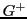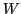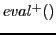## Calculating the UnknownIn the real world is the success setnot known. One has to 'approach' this unknown set by 'searching' it. If we assume the genotype as some n-dimensional coordinates in a n-dimensional space then are the values of the real evaluation functionsome additional spacewhich includes the worldas a parameter. Because the real worldcan change through time we have to assume that the 'ideal' success set is not necessarily a 'fixed' set; the ideal success set can change too! For some finiteduration one can assume that the success setis stable and the evaluation functionis then an empirical estimate of the evaluation function.

Gerd Doeben-Henisch 2013-01-14